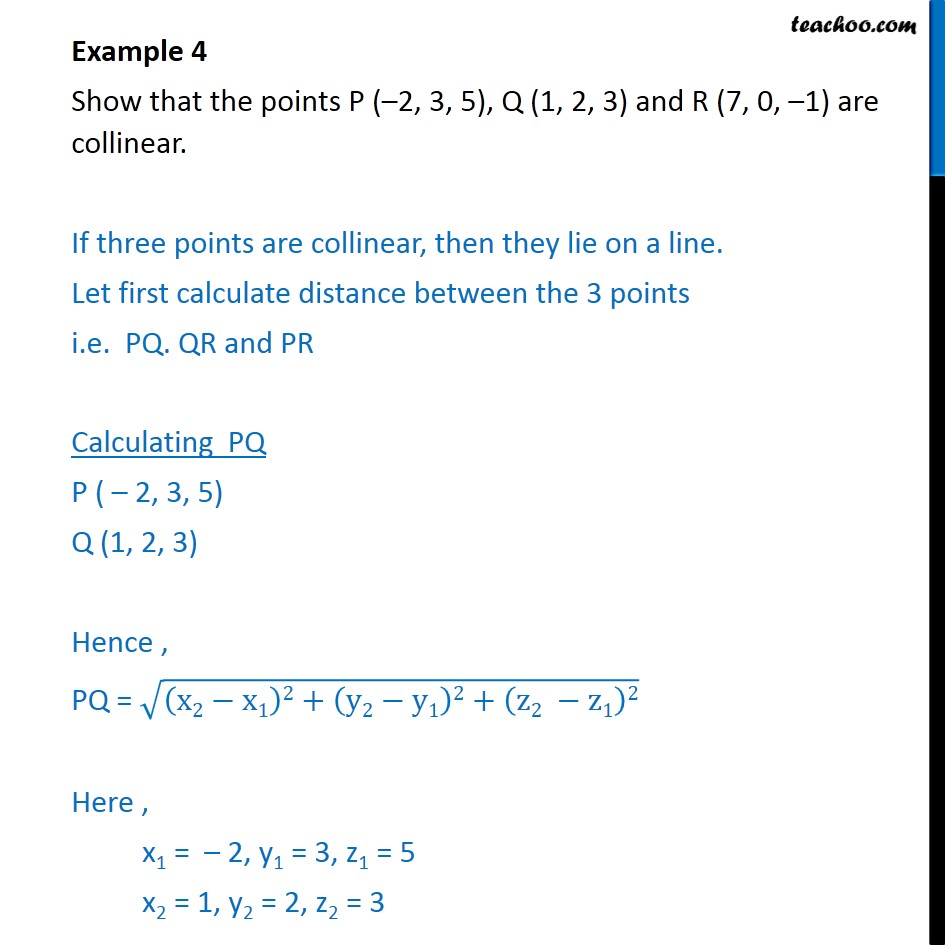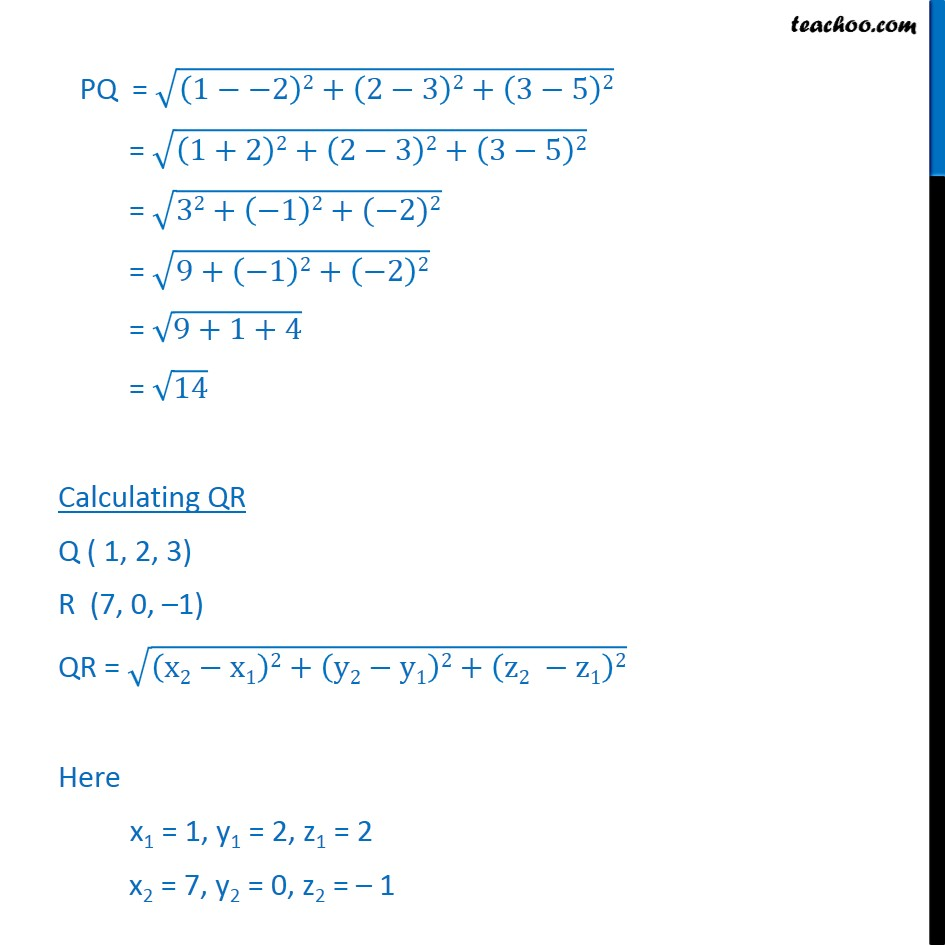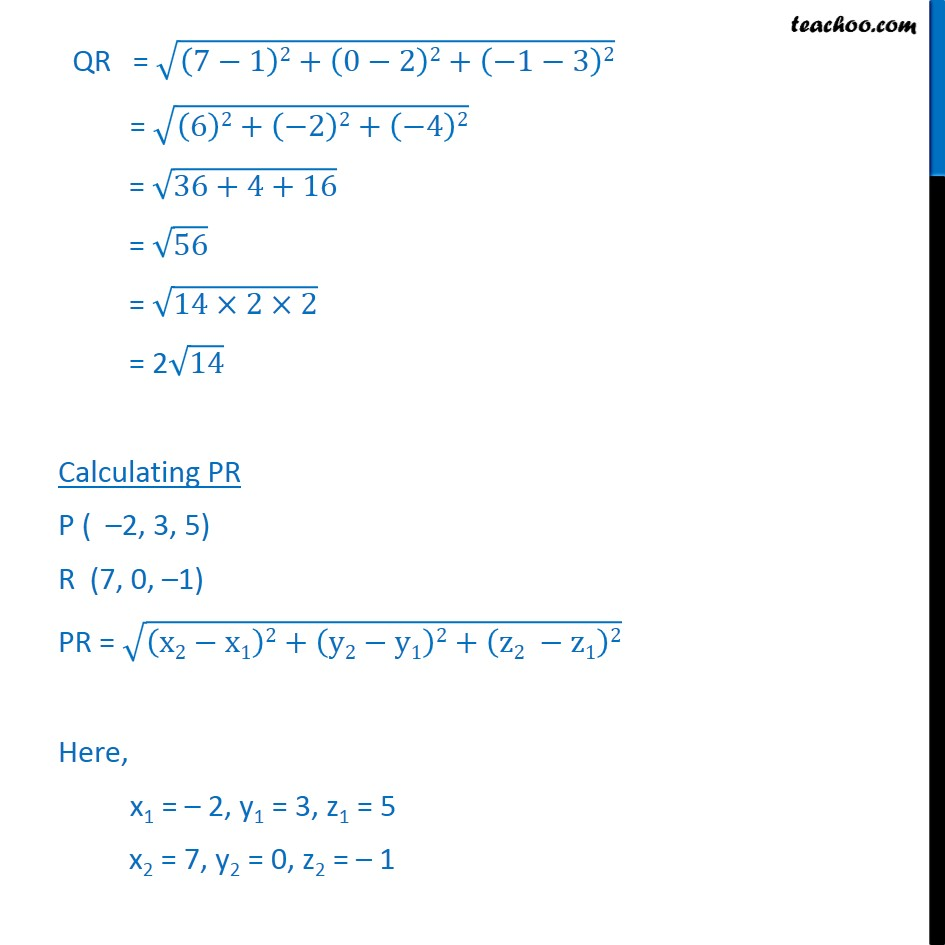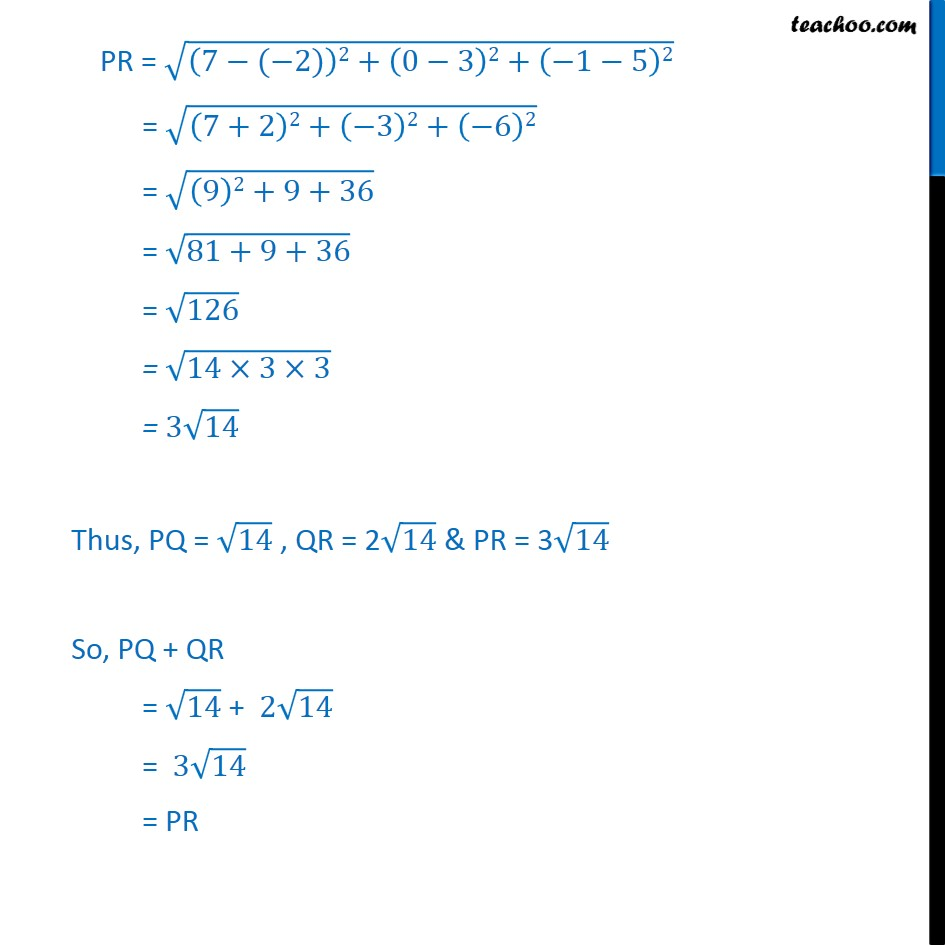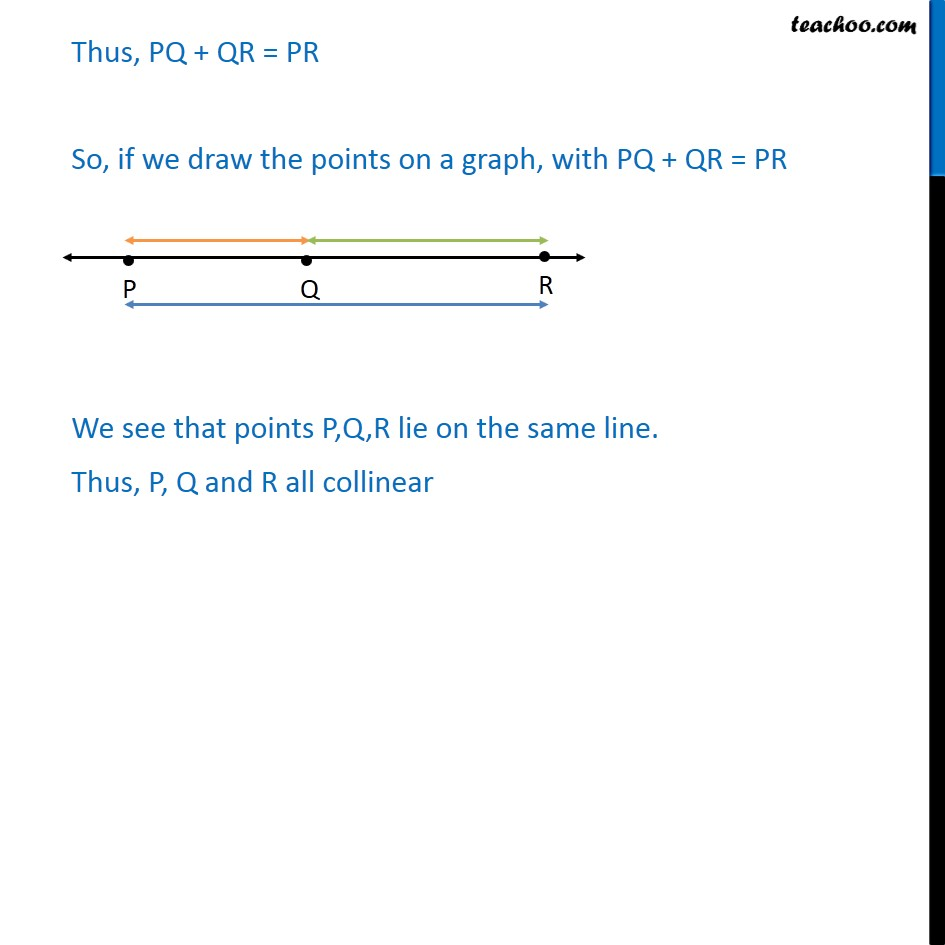1. Chapter 12 Class 11 Introduction to Three Dimensional Geometry
2. Serial order wise
3. Examples

Transcript

Example 4 Show that the points P ( 2, 3, 5), Q (1, 2, 3) and R (7, 0, 1) are collinear. If three points are collinear, then they lie on a line. Let first calculate distance between the 3 points i.e. PQ. QR and PR Calculating PQ P ( 2, 3, 5) Q (1, 2, 3) Hence , PQ = x2 x1 2+ y2 y1 2+ z2 z1 2 Here , x1 = 2, y1 = 3, z1 = 5 x2 = 1, y2 = 2, z2 = 3 PQ = 1 2 2+ 2 3 2+ 3 5 2 = 1+2 2+ 2 3 2+ 3 5 2 = 32+ 1 2+( 2)2 = 9+ 1 2+ 2 2 = 9+1+4 = 14 Calculating QR Q ( 1, 2, 3) R (7, 0, 1) QR = x2 x1 2+ y2 y1 2+ z2 z1 2 Here x1 = 1, y1 = 2, z1 = 2 x2 = 7, y2 = 0, z2 = 1 QR = 7 1 2+ 0 2 2+ 1 3 2 = 6 2+ 2 2+ 4 2 = 36+4+16 = 56 = 14 2 2 = 2 14 Calculating PR P ( 2, 3, 5) R (7, 0, 1) PR = x2 x1 2+ y2 y1 2+ z2 z1 2 Here, x1 = 2, y1 = 3, z1 = 5 x2 = 7, y2 = 0, z2 = 1 PR = 7 ( 2) 2+ 0 3 2+ 1 5 2 = 7+2 2+ 3 2+ 6 2 = 9 2+9+36 = 81+9+36 = 126 = 14 3 3 = 3 14 Thus, PQ = 14 , QR = 2 14 & PR = 3 14 So, PQ + QR = 14 + 2 14 = 3 14 = PR Thus, PQ + QR = PR So, if we draw the points on a graph, with PQ + QR = PR We see that points P,Q,R lie on the same line. Thus, P, Q and R all collinear

Examples